# Extrema of f(x1,x2) VI

## LabVIEW 2018 Help

Edition Date: March 2018
Part Number: 371361R-01
View Product InfoLabVIEW 2015 HelpLabVIEW 2016 HelpLabVIEW 2017 HelpLabVIEW 2018 HelpLabVIEW 2019 Help

Owning Palette: Calculus VIs

Requires: Full Development System

The algorithm looks for local extrema of a given function of two variables on a given rectangle. The absolute distance between two extrema must be equal to or greater than 1E–6 for the two vectors to register as different from each other.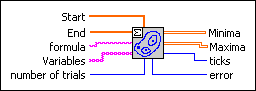Start specifies the start points of both variables, that is, an array of length 2. The default values are (0, 0).End specifies the end points of both variables, that is, an array of length 2. The default values are (1, 1).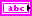formula is a string describing the function. The formula can contain any number of valid variables.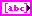Variables is an array of two strings representing the two variables with respect to the naming conventions of the Formula Parsing VIs. The default variables are (x1, x2).number of trials is the number of randomly chosen 2D starting points of the algorithm.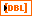Minima is a 2D array of all local minima of the given function. Any local minimum is presented by two coordinates.Maxima is a 2D array of all local maxima of the given function. Any local maximum is presented by two coordinates.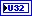ticks is the time effort for the whole calculation of the function values in milliseconds.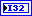error returns any error or warning from the VI. You can wire error to the Error Cluster From Error Code VI to convert the error code or warning into an error cluster.

## Extrema of f(x1,x2) Details

While the number of trials can be very large, there is no guarantee you can find all or at least one zero, local minimum or local maximum of the given function. Moreover, such points do not exist in all cases.

Though the randomly chosen start points of the extrema algorithm belong to the initially given rectangle, sometimes the determined extrema can be found outside of the rectangle. In this case, these values will also be presented.